### Home > A2C > Chapter 13 > Lesson 13.2.2 > Problem13-122

13-122.
1. Solve for x, correct to three decimal places. Homework Help ✎

1. 27 = 6x

2.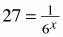3.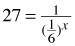4. Show that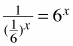.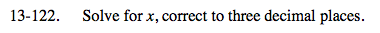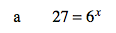x ≈ 1.839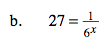x ≈ −1.839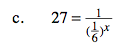$\left( \frac{1}{6}\right)^x=\frac{1}{27}$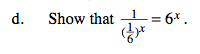Try rewriting the expression with negative exponents instead of fractions.